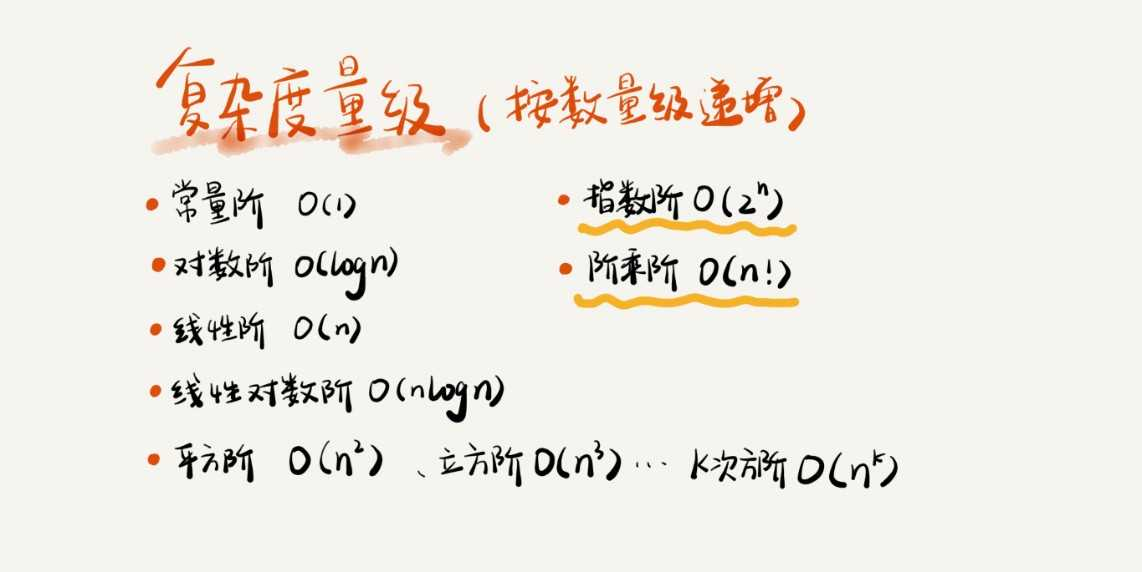# 002-数据结构与算法-复杂度分析

## 时间复杂度

### 计算方式

1 int cal(int n) {
2   int sum = 0;
3   int i = 1;
4   for (; i <= n; ++i) {
5     sum = sum + i;
6   }
7   return sum;
8 }


### 最好/最坏/平均时间复杂度

// n 表示数组 array 的长度
1 int find(int[] array, int n, int x) {
2  int i = 0;
3  int pos = -1;
4  for (; i < n; ++i) {
5    if (array[i] == x) {
6        pos = i;
7        break;
8     }
11  }
12  return pos;
13 }


### 常见的时间复杂度## 空间复杂度

### 计算方式

1 void print(int n) {
2  int i = 0;
3  int[] a = new int[n];
4  for (i; i <n; ++i) {
5    a[i] = i * i;
6  }

7  for (i = n-1; i >= 0; --i) {
8    print out a[i]
9  }
10}